# Review Conversion Formula of particles of Particles Moles

• Slides: 28Review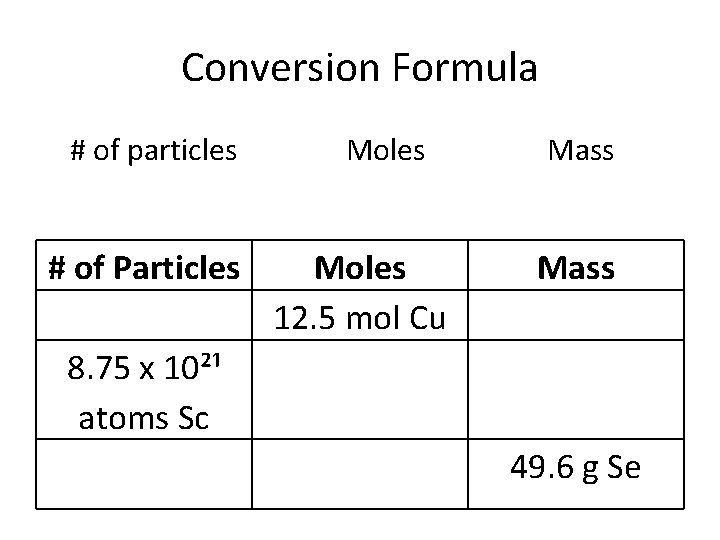Conversion Formula # of particles # of Particles Moles 12. 5 mol Cu Mass 8. 75 x 10²¹ atoms Sc 49. 6 g SeMoles of Compounds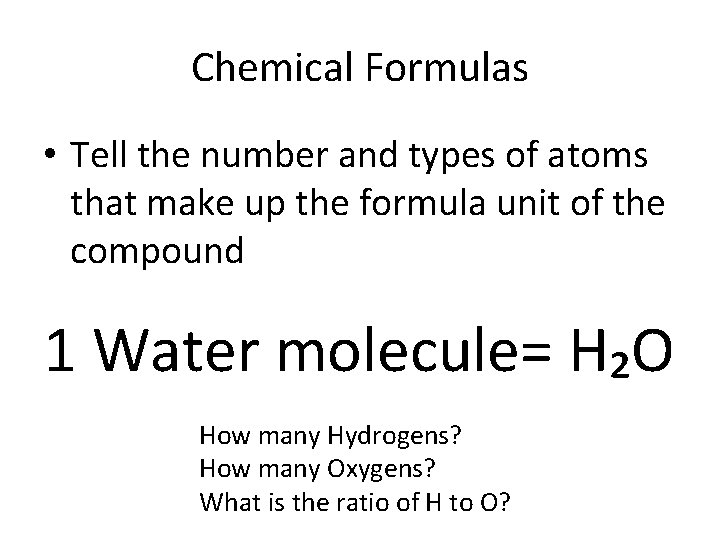Chemical Formulas • Tell the number and types of atoms that make up the formula unit of the compound 1 Water molecule= H₂O How many Hydrogens? How many Oxygens? What is the ratio of H to O?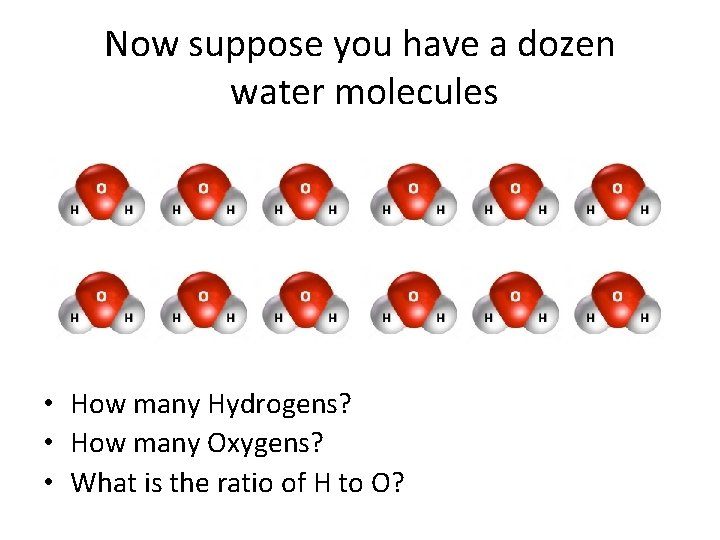Now suppose you have a dozen water molecules • How many Hydrogens? • How many Oxygens? • What is the ratio of H to O?What if you have 1 mol of water molecules? • How many Hydrogens? • How many Oxygens? • What is the ratio of H to O?• Chemical formulas tell how many moles of each element are in 1 mole of that molecule or formula unit How many moles? C₄H₁₀ = Cu. SO₄ = Al(OH)₃ =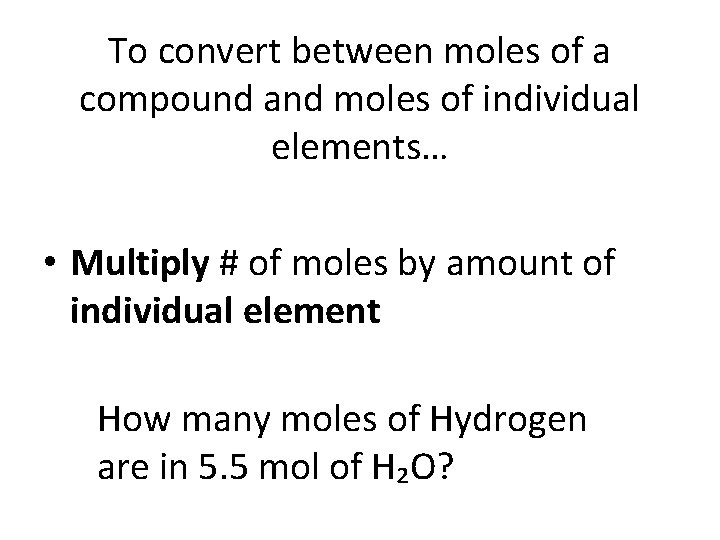To convert between moles of a compound and moles of individual elements… • Multiply # of moles by amount of individual element How many moles of Hydrogen are in 5. 5 mol of H₂O?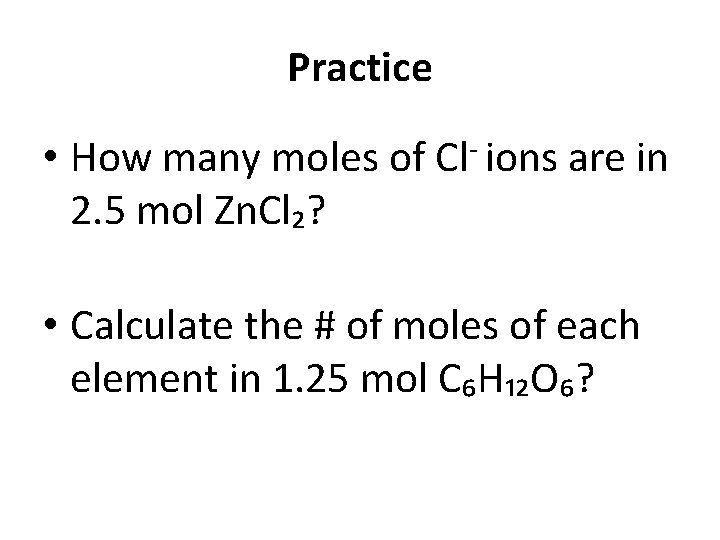Practice • How many moles of Cl- ions are in 2. 5 mol Zn. Cl₂? • Calculate the # of moles of each element in 1. 25 mol C₆H₁₂O₆?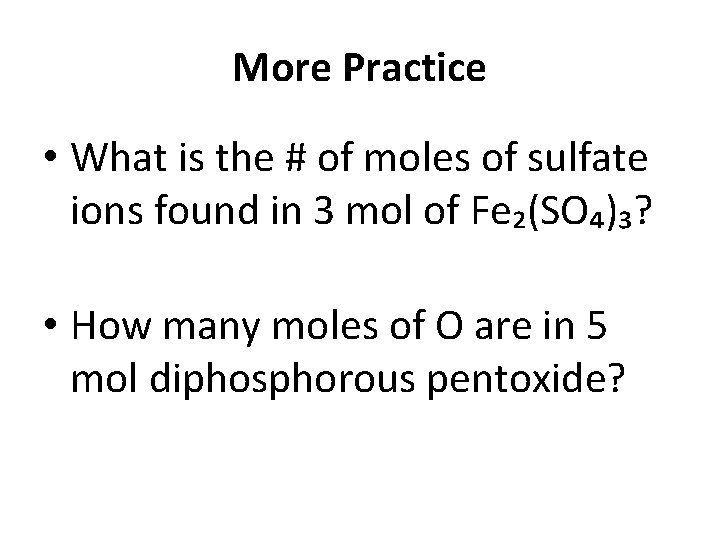More Practice • What is the # of moles of sulfate ions found in 3 mol of Fe₂(SO₄)₃? • How many moles of O are in 5 mol diphosphorous pentoxide?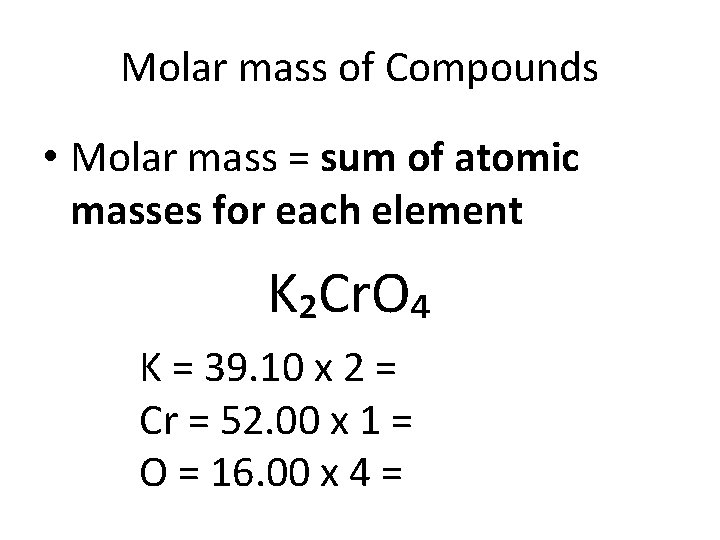Molar mass of Compounds • Molar mass = sum of atomic masses for each element K₂Cr. O₄ K = 39. 10 x 2 = Cr = 52. 00 x 1 = O = 16. 00 x 4 =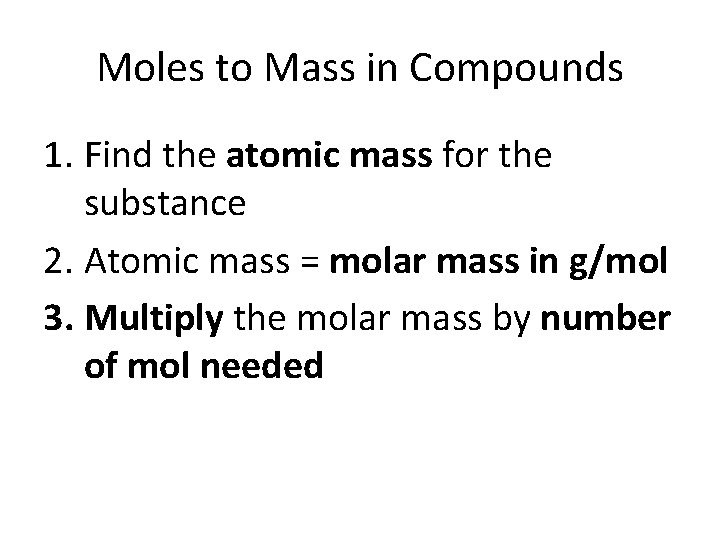Moles to Mass in Compounds 1. Find the atomic mass for the substance 2. Atomic mass = molar mass in g/mol 3. Multiply the molar mass by number of mol needed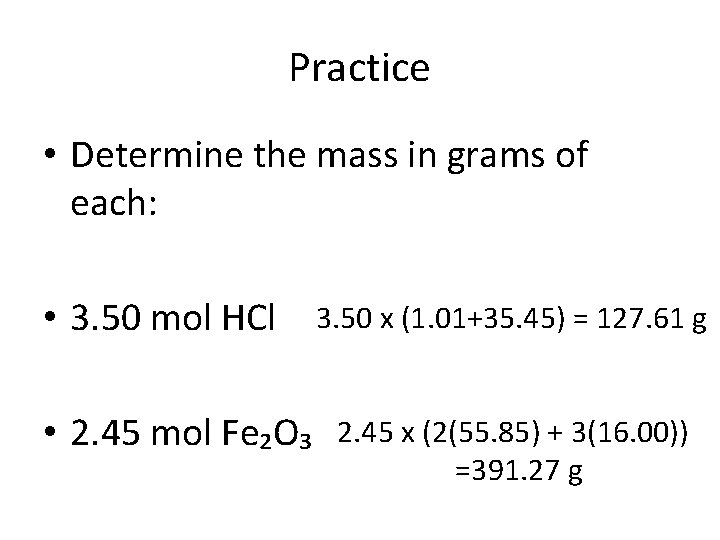Practice • Determine the mass in grams of each: • 3. 50 mol HCl • 2. 45 mol Fe₂O₃ 3. 50 x (1. 01+35. 45) = 127. 61 g 2. 45 x (2(55. 85) + 3(16. 00)) =391. 27 g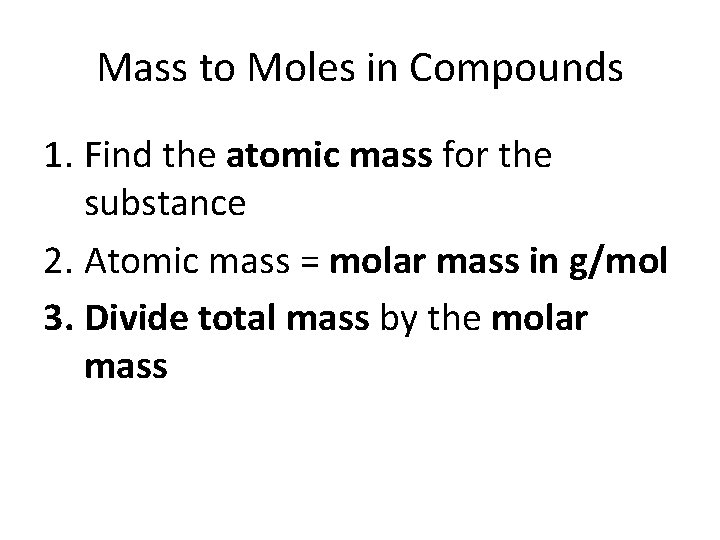Mass to Moles in Compounds 1. Find the atomic mass for the substance 2. Atomic mass = molar mass in g/mol 3. Divide total mass by the molar massPractice • How many moles of Ti. O₂ are in 43. 5 g? • Molar mass = 47. 87 + 2(16. 00) = 79. 87 • Total mass/molar mass 43. 5/79. 87 = 0. 545 mol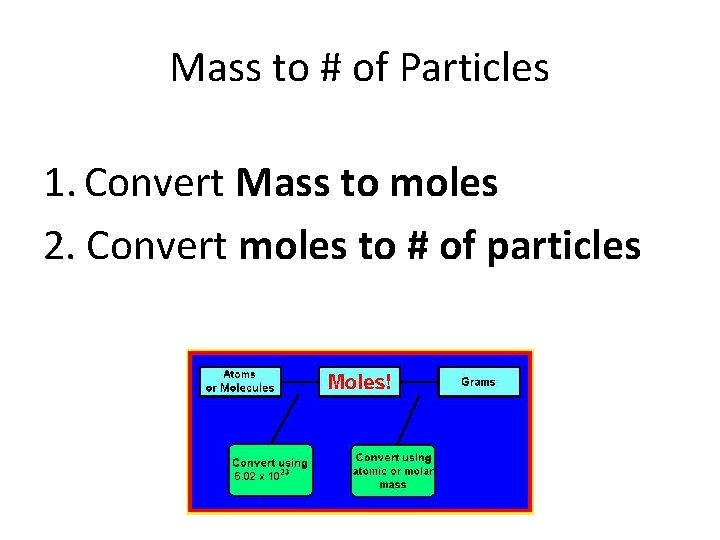Mass to # of Particles 1. Convert Mass to moles 2. Convert moles to # of particles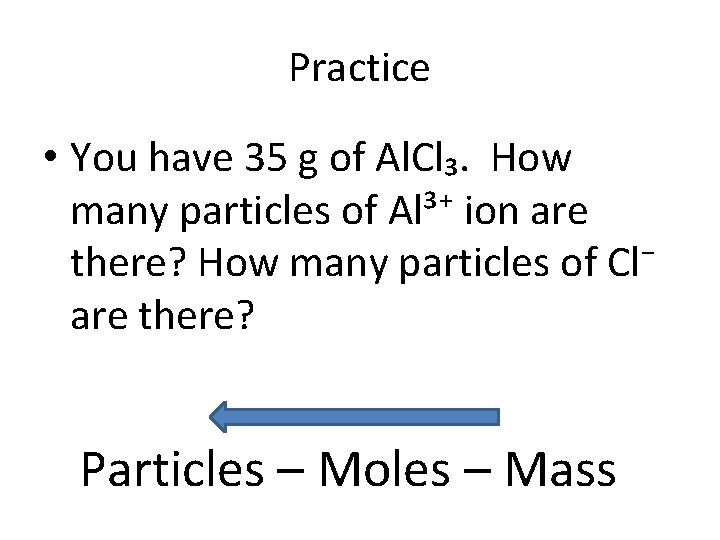Practice • You have 35 g of Al. Cl₃. How many particles of Al³⁺ ion are there? How many particles of Cl⁻ are there? Particles – Moles – Mass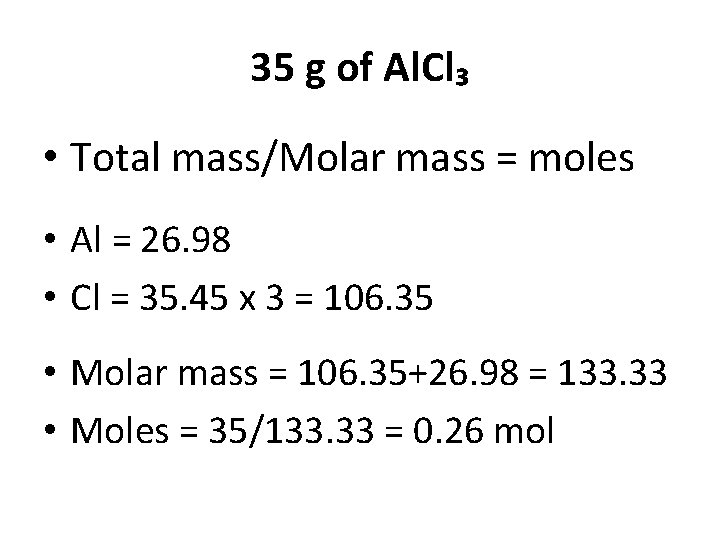35 g of Al. Cl₃ • Total mass/Molar mass = moles • Al = 26. 98 • Cl = 35. 45 x 3 = 106. 35 • Molar mass = 106. 35+26. 98 = 133. 33 • Moles = 35/133. 33 = 0. 26 mol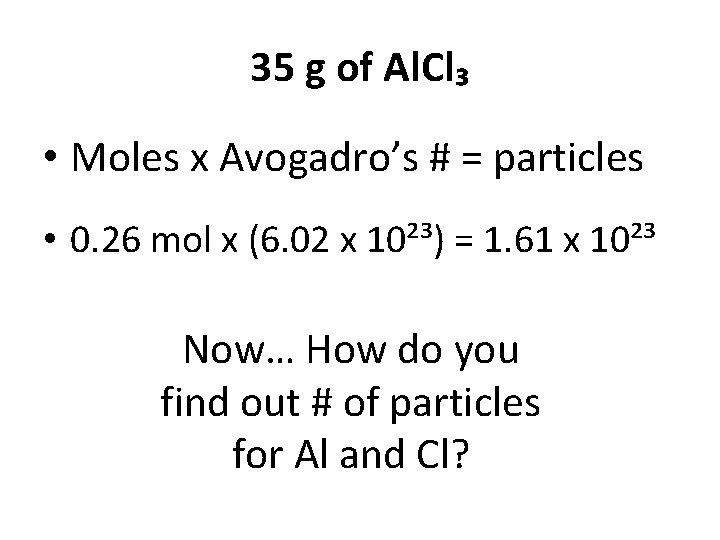35 g of Al. Cl₃ • Moles x Avogadro’s # = particles • 0. 26 mol x (6. 02 x 10²³) = 1. 61 x 10²³ Now… How do you find out # of particles for Al and Cl?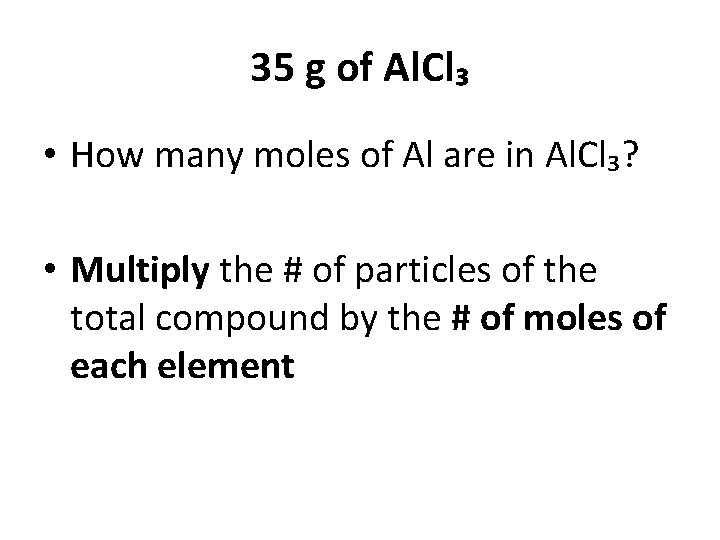35 g of Al. Cl₃ • How many moles of Al are in Al. Cl₃? • Multiply the # of particles of the total compound by the # of moles of each element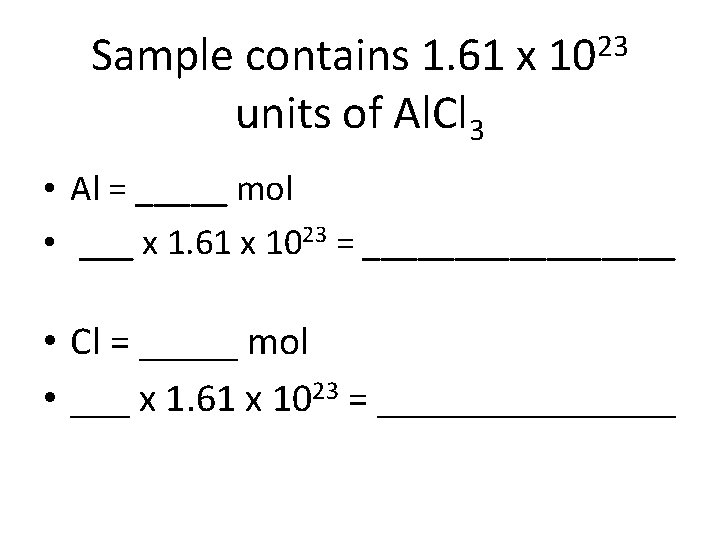Sample contains 1. 61 x units of Al. Cl 3 23 10 • Al = _____ mol • ___ x 1. 61 x 1023 = _________ • Cl = _____ mol • ___ x 1. 61 x 1023 = ________To find the mass of 1 formula unit of a compound… • Divide molar mass of the compound by Avogadro’s # • Molar mass of Al. Cl 3 is 133. • 133. 33/6. 02 x 1023 = 2. 21 x 10⁻²²Practice Worksheet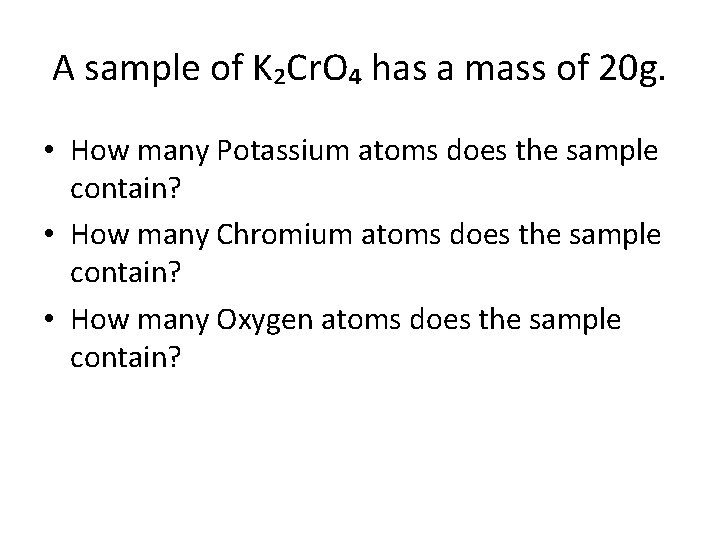A sample of K₂Cr. O₄ has a mass of 20 g. • How many Potassium atoms does the sample contain? • How many Chromium atoms does the sample contain? • How many Oxygen atoms does the sample contain?#particles moles • Find the molar mass of the whole compound K₂Cr. O₄ K = 39. 10 x 2 = 78. 2 Cr = 52. 00 x 1 = 52 O = 16. 00 x 4 = 64 mass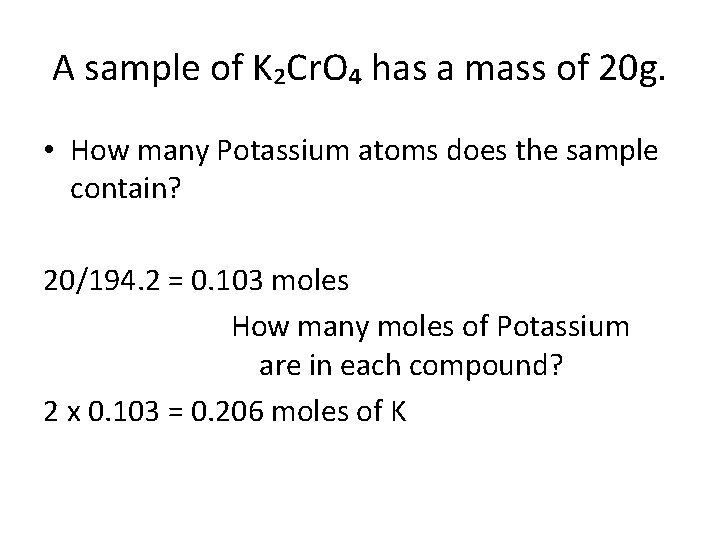A sample of K₂Cr. O₄ has a mass of 20 g. • How many Potassium atoms does the sample contain? 20/194. 2 = 0. 103 moles How many moles of Potassium are in each compound? 2 x 0. 103 = 0. 206 moles of K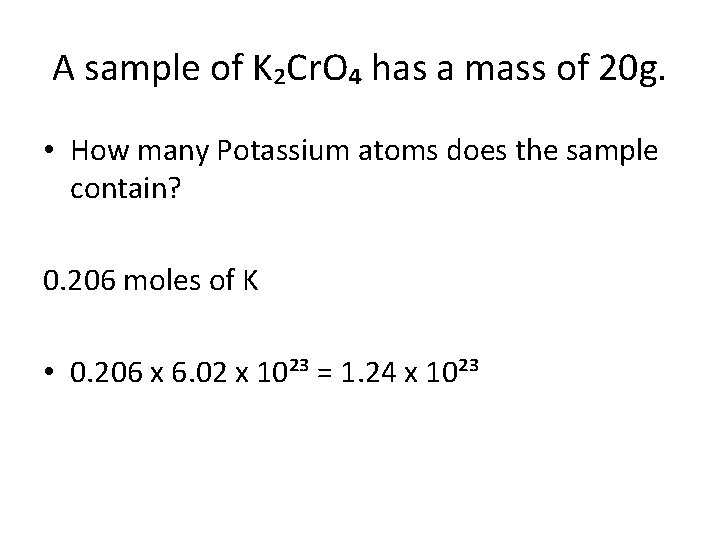A sample of K₂Cr. O₄ has a mass of 20 g. • How many Potassium atoms does the sample contain? 0. 206 moles of K • 0. 206 x 6. 02 x 10²³ = 1. 24 x 10²³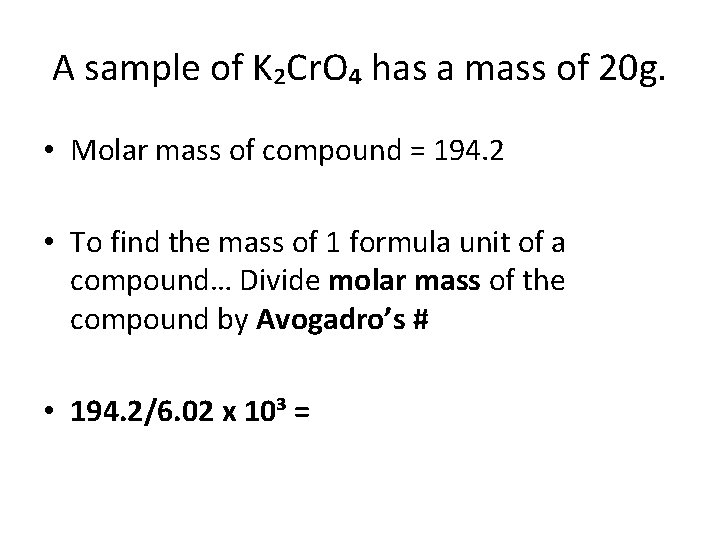A sample of K₂Cr. O₄ has a mass of 20 g. • Molar mass of compound = 194. 2 • To find the mass of 1 formula unit of a compound… Divide molar mass of the compound by Avogadro’s # • 194. 2/6. 02 x 10³ =